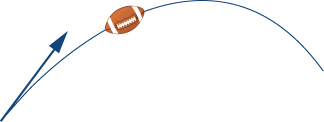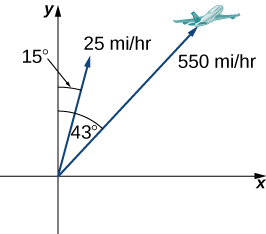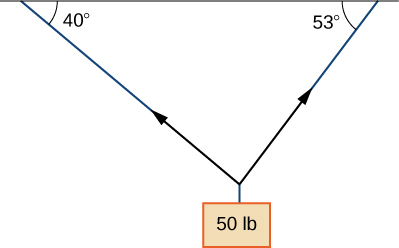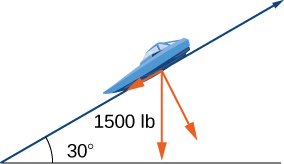$$\newcommand{\id}{\mathrm{id}}$$ $$\newcommand{\Span}{\mathrm{span}}$$ $$\newcommand{\kernel}{\mathrm{null}\,}$$ $$\newcommand{\range}{\mathrm{range}\,}$$ $$\newcommand{\RealPart}{\mathrm{Re}}$$ $$\newcommand{\ImaginaryPart}{\mathrm{Im}}$$ $$\newcommand{\Argument}{\mathrm{Arg}}$$ $$\newcommand{\norm}{\| #1 \|}$$ $$\newcommand{\inner}{\langle #1, #2 \rangle}$$ $$\newcommand{\Span}{\mathrm{span}}$$

# 7.1E: Excersies

$$\newcommand{\vecs}{\overset { \rightharpoonup} {\mathbf{#1}} }$$ $$\newcommand{\vecd}{\overset{-\!-\!\rightharpoonup}{\vphantom{a}\smash {#1}}}$$$$\newcommand{\id}{\mathrm{id}}$$ $$\newcommand{\Span}{\mathrm{span}}$$ $$\newcommand{\kernel}{\mathrm{null}\,}$$ $$\newcommand{\range}{\mathrm{range}\,}$$ $$\newcommand{\RealPart}{\mathrm{Re}}$$ $$\newcommand{\ImaginaryPart}{\mathrm{Im}}$$ $$\newcommand{\Argument}{\mathrm{Arg}}$$ $$\newcommand{\norm}{\| #1 \|}$$ $$\newcommand{\inner}{\langle #1, #2 \rangle}$$ $$\newcommand{\Span}{\mathrm{span}}$$ $$\newcommand{\id}{\mathrm{id}}$$ $$\newcommand{\Span}{\mathrm{span}}$$ $$\newcommand{\kernel}{\mathrm{null}\,}$$ $$\newcommand{\range}{\mathrm{range}\,}$$ $$\newcommand{\RealPart}{\mathrm{Re}}$$ $$\newcommand{\ImaginaryPart}{\mathrm{Im}}$$ $$\newcommand{\Argument}{\mathrm{Arg}}$$ $$\newcommand{\norm}{\| #1 \|}$$ $$\newcommand{\inner}{\langle #1, #2 \rangle}$$ $$\newcommand{\Span}{\mathrm{span}}$$

## Exercise $$\PageIndex{1}$$

For the following exercises, consider points $$P(−1,3), Q(1,5),$$ and $$R(−3,7)$$. Determine the requested vectors and express each of them a. in component form and b. by using the standard unit vectors.

1) $$\vec{PQ}$$

$$a. \vec{PQ}=⟨2,2⟩; b. \vec{PQ}=2i+2j$$

2) $$\vec{PR}$$

3) $$\vec{QP}$$

$$a. \vec{QP}=⟨−2,−2⟩; b. \vec{QP}=−2i−2j$$

4) $$\vec{RP}$$

5) $$\vec{PQ}+\vec{PR}$$

$$a. \vec{PQ}+\vec{PR}=⟨0,6⟩; b. \vec{PQ}+\vec{PR}=6j$$

6) $$\vec{PQ}−\vec{PR}$$

7) $$2\vec{PQ}−2\vec{PR}$$

$$a. 2\vec{PQ}→−2\vec{PR}=⟨8,−4⟩; b. 2\vec{PQ}−2\vec{PR}=8i−4j$$

8) $$2\vec{PQ}+\frac{1}{2}\vec{PR}$$

9) The unit vector in the direction of $$\vec{PQ}$$

$$a. ⟨\frac{1}{\sqrt{2}},\frac{1}{\sqrt{2}}⟩; b. \frac{1}{\sqrt{2}}i+\frac{1}{\sqrt{2}}j$$

10) The unit vector in the direction of $$\vec{PR}$$

11) A vector $$v$$ has initial point $$(−1,−3)$$ and terminal point $$(2,1)$$. Find the unit vector in the direction of $$v$$. Express the answer in component form.

$$⟨\frac{3}{5},\frac{4}{5}⟩$$

12) A vector $$v$$ has initial point $$(−2,5)$$ and terminal point $$(3,−1)$$. Find the unit vector in the direction of $$v$$. Express the answer in component form.

13) The vector $$v$$ has an initial point $$P(1,0)$$ and terminal point $$Q$$ that is on the y-axis and above the initial point. Find the coordinates of terminal point $$Q$$ such that the magnitude of the vector $$v$$ is $$\sqrt{5}$$.

$$Q(0,2)$$

14) The vector $$v$$ has an initial point $$P(1,1)$$ and terminal point $$Q$$ that is on the x-axis and left of the initial point. Find the coordinates of terminal point $$Q$$ such that the magnitude of the vector $$v$$ is $$\sqrt{10}$$.

## Exercise $$\PageIndex{2}$$

For the following exercises, use the given vectors $$a$$ and $$b$$.

a. Determine the vector sum $$a+b$$ and express it in both the component form and by using the standard unit vectors.

b. Find the vector difference $$a−b$$ and express it in both the component form and by using the standard unit vectors.

c. Verify that the vectors $$a, b,$$ and $$a+b$$, and, respectively, $$a, b$$, and $$a−b$$ satisfy the triangle inequality.

d. Determine the vectors $$2a, −b,$$ and $$2a−b.$$ Express the vectors in both the component form and by using standard unit vectors.

15) $$a=2i+j, b=i+3j$$

$$a. a+b=3i+4j, a+b=⟨3,4⟩;$$ b. $$a−b=i−2j, a−b=⟨1,−2⟩;$$ c. Answers will vary; d. $$2a=4i+2j, 2a=⟨4,2⟩, −b=−i−3j, −b=⟨−1,−3⟩, 2a−b=3i−j, 2a−b=⟨3,−1⟩$$

16) $$a=2i, b=−2i+2j$$

17) Let $$a$$ be a standard-position vector with terminal point $$(−2,−4)$$. Let $$b$$ be a vector with initial point $$(1,2)$$ and terminal point $$(−1,4)$$. Find the magnitude of vector $$−3a+b−4i+j.$$

$$15$$

18) Let $$a$$ be a standard-position vector with terminal point at $$(2,5)$$. Let $$b$$ be a vector with initial point $$(−1,3)$$ and terminal point $$(1,0)$$. Find the magnitude of vector $$a−3b+14i−14j.$$

## Exercise $$\PageIndex{3}$$

19) Let $$u$$ and $$v$$ be two nonzero vectors that are nonequivalent. Consider the vectors $$a=4u+5v$$ and $$b=u+2v$$ defined in terms of $$u$$ and $$v$$. Find the scalar $$λ$$ such that vectors $$a+λb$$ and $$u−v$$ are equivalent.

$$λ=−3$$

20) Let $$u$$ and $$v$$ be two nonzero vectors that are nonequivalent. Consider the vectors $$a=2u−4v$$ and $$b=3u−7v$$ defined in terms of $$u$$ and $$v$$. Find the scalars $$α$$ and $$β$$ such that vectors $$αa+βb$$ and $$u−v$$ are equivalent.

## Exercise $$\PageIndex{4}$$

21) Consider the vector $$a(t)=⟨cost,sint⟩$$ with components that depend on a real number $$t$$. As the number $$t$$ varies, the components of $$a(t)$$ change as well, depending on the functions that define them.

a. Write the vectors $$a(0)$$ and $$a(π)$$ in component form.

b. Show that the magnitude $$∥a(t)∥$$ of vector $$a(t)$$ remains constant for any real number $$t$$.

c. As $$t$$ varies, show that the terminal point of vector $$a(t)$$ describes a circle centered at the origin of radius $$1$$.

$$a. a(0)=⟨1,0⟩, a(π)=⟨−1,0⟩;$$ b. Answers may vary; c. Answers may vary

22) Consider vector $$a(x)=⟨x,\sqrt{1−x^2}⟩$$ with components that depend on a real number $$x∈[−1,1]$$. As the number $$x$$ varies, the components of $$a(x)$$ change as well, depending on the functions that define them.

a. Write the vectors $$a(0)$$ and $$a(1)$$ in component form.

b. Show that the magnitude $$∥a(x)∥$$ of vector $$a(x)$$ remains constant for any real number $$x$$

c. As $$x$$ varies, show that the terminal point of vector $$a(x)$$ describes a circle centered at the origin of radius $$1$$.

23) Show that vectors $$a(t)=⟨cost,sint⟩$$ and $$a(x)=⟨x,\sqrt{1−x^2}⟩$$ are equivalent for $$x=r$$ and $$t=2kπ$$, where $$k$$ is an integer.

24) Show that vectors $$a(t)=⟨cost,sint⟩$$ and $$a(x)=⟨x,\sqrt{1−x^2}⟩$$ are opposite for $$x=r$$ and $$t=π+2kπ$$, where $$k$$ is an integer.

## Exercise $$\PageIndex{5}$$

For the following exercises, find vector $$v$$ with the given magnitude and in the same direction as vector $$u$$.

25) $$‖v‖=7,u=⟨3,4⟩$$

$$v=⟨\frac{21}{5},\frac{28}{5}⟩$$

26) $$‖v‖=3,u=⟨−2,5⟩$$

27) $$‖v‖=7,u=⟨3,−5⟩$$

$$v=⟨\frac{21\sqrt{34}}{34},−\frac{35\sqrt{34}}{34}⟩$$

28) $$‖v‖=10,u=⟨2,−1⟩$$

## Exercise $$\PageIndex{6}$$

For the following exercises, find the component form of vector $$u$$, given its magnitude and the angle the vector makes with the positive x-axis. Give exact answers when possible.

29) $$‖u‖=2, θ=30°$$

$$u=⟨\sqrt{3},1⟩$$

30) $$‖u‖=6, θ=60°$$

31) $$‖u‖=5, θ=\frac{π}{2}$$

$$u=⟨0,5⟩$$

32) $$‖u‖=8, θ=π$$

33) $$‖u‖=10, θ=\frac{5π}{6}$$

$$u=⟨−5\sqrt{3},5⟩$$

34) $$‖u‖=50, θ=\frac{3π}{4}$$

## Exercise $$\PageIndex{7}$$

For the following exercises, find the component form of vector $$u$$, given its magnitude and the angle the vector makes with the positive x-axis. Give exact answers when possible.

29) $$‖u‖=2, θ=30°$$

$$u=⟨\sqrt{3},1⟩$$

30) $$‖u‖=6, θ=60°$$

31) $$‖u‖=5, θ=\frac{π}{2}$$

$$u=⟨0,5⟩$$

32) $$‖u‖=8, θ=π$$

33) $$‖u‖=10, θ=\frac{5π}{6}$$

$$u=⟨−5\sqrt{3},5⟩$$

34) $$‖u‖=50, θ=\frac{3π}{4}$$

## Exercise $$\PageIndex{8}$$

For the following exercises, vector $$u$$ is given. Find the angle $$θ∈[0,2π)$$ that vector $$u$$ makes with the positive direction of the x-axis, in a counter-clockwise direction.

35) $$u=5\sqrt{2}i−5\sqrt{2}j$$

$$θ=\frac{7π}{4}$$

36) $$u=−\sqrt{3}i−j$$

37) Let $$a=⟨a_1,a_2⟩, b=⟨b_1,b_2⟩$$, and $$c=⟨c_1,c_2⟩$$ be three nonzero vectors. If $$a_1b_2−a_2b_1≠0$$, then show there are two scalars, $$α$$ and $$β$$, such that $$c=αa+βb.$$

## Exercise $$\PageIndex{9}$$

38) Consider vectors $$a=⟨2,−4⟩, b=⟨−1,2⟩,$$ and $$0$$ Determine the scalars $$α$$ and $$β$$ such that $$c=αa+βb$$.

39) Let $$P(x_0,f(x_0))$$ be a fixed point on the graph of the differential function $$f$$ with a domain that is the set of real numbers.

a. Determine the real number $$z_0$$ such that point $$Q(x_0+1,z_0)$$ is situated on the line tangent to the graph of $$f$$ at point $$P$$.

b. Determine the unit vector $$u$$ with initial point $$P$$ and terminal point $$Q$$.

$$a. z_0=f(x_0)+f′(x_0); b. u=\frac{1}{\sqrt{1+[f′(x_0)]^2}}⟨1,f′(x_0)⟩$$

40) Consider the function $$f(x)=x^4,$$ where $$x∈R$$.

a. Determine the real number $$z_0$$ such that point $$Q(2,z_0)$$ s situated on the line tangent to the graph of $$f$$ at point $$P(1,1)$$.

b. Determine the unit vector $$u$$ with initial point $$P$$ and terminal point $$Q$$.

41) Consider $$f$$ and $$g$$ two functions defined on the same set of real numbers $$D$$. Let $$a=⟨x,f(x)⟩$$ and $$b=⟨x,g(x)⟩$$ be two vectors that describe the graphs of the functions, where $$x∈D$$. Show that if the graphs of the functions $$f$$ and $$g$$ do not intersect, then the vectors $$a$$ and $$b$$ are not equivalent.

42) Find $$x∈R$$ such that vectors $$a=⟨x,sinx⟩$$ and $$b=⟨x,cosx⟩$$ are equivalent.

43) Calculate the coordinates of point $$D$$ such that $$ABCD$$ is a parallelogram, with $$A(1,1), B(2,4)$$, and $$C(7,4)$$.

$$D(6,1)$$

44) Consider the points $$A(2,1), B(10,6), C(13,4), and D(16,−2).$$ Determine the component form of vector $$\vec{AD}$$.

## Exercise $$\PageIndex{10}$$

45) The speed of an object is the magnitude of its related velocity vector. A football thrown by a quarterback has an initial speed of $$70$$ mph and an angle of elevation of $$30°$$. Determine the velocity vector in mph and express it in component form. (Round to two decimal places.)$$⟨60.62,35⟩$$

46) A baseball player throws a baseball at an angle of $$30°$$ with the horizontal. If the initial speed of the ball is $$100$$ mph, find the horizontal and vertical components of the initial velocity vector of the baseball. (Round to two decimal places.)

47) A bullet is fired with an initial velocity of $$1500$$ ft/sec at an angle of $$60°$$ with the horizontal. Find the horizontal and vertical components of the velocity vector of the bullet. (Round to two decimal places.)The horizontal and vertical components are $$750$$ ft/sec and $$1299.04$$ ft/sec, respectively.

48) [T] A 65-kg sprinter exerts a force of $$798$$ N at a $$19°$$ angle with respect to the ground on the starting block at the instant a race begins. Find the horizontal component of the force. (Round to two decimal places.)

49) [T] Two forces, a horizontal force of $$45$$ lb and another of $$52$$ lb, act on the same object. The angle between these forces is $$25°$$. Find the magnitude and direction angle from the positive x-axis of the resultant force that acts on the object. (Round to two decimal places.)The magnitude of the resultant force is $$94.71$$ lb; the direction angle is $$13.42°$$.

50) [T] Two forces, a vertical force of $$26$$ lb and another of $$45$$ lb, act on the same object. The angle between these forces is $$55°$$. Find the magnitude and direction angle from the positive x-axis of the resultant force that acts on the object. (Round to two decimal places.)

51) [T] Three forces act on object. Two of the forces have the magnitudes $$58$$ N and $$27$$ N, and make angles $$53°$$ and $$152°$$, respectively, with the positive x-axis. Find the magnitude and the direction angle from the positive x-axis of the third force such that the resultant force acting on the object is zero. (Round to two decimal places.)

The magnitude of the third vector is $$60.03$$N; the direction angle is $$259.38°$$.

52) Three forces with magnitudes 80 lb, 120 lb, and 60 lb act on an object at angles of $$45°, 60°$$ and $$30°$$, respectively, with the positive x-axis. Find the magnitude and direction angle from the positive x-axis of the resultant force. (Round to two decimal places.)53) [T] An airplane is flying in the direction of $$43°$$ east of north (also abbreviated as $$N43E$$) at a speed of $$550$$ mph. A wind with speed $$25$$ mph comes from the southwest at a bearing of $$N15E$$. What are the ground speed and new direction of the airplane?The new ground speed of the airplane is $$572.19$$ mph; the new direction is $$N41.82E.$$

54) [T] A boat is travelling in the water at $$30$$ mph in a direction of $$N20E$$ (that is, $$20°$$ east of north). A strong current is moving at $$15$$ mph in a direction of $$N45E$$. What are the new speed and direction of the boat?55) [T] A 50-lb weight is hung by a cable so that the two portions of the cable make angles of $$40°$$ and $$53°$$, respectively, with the horizontal. Find the magnitudes of the forces of tension $$T_1$$ and $$T_2$$ in the cables if the resultant force acting on the object is zero. (Round to two decimal places.)$$∥T_1∥=30.13lb, ∥T_2∥=38.35lb$$

56) [T] A 62-lb weight hangs from a rope that makes the angles of $$29°$$ and $$61°$$, respectively, with the horizontal. Find the magnitudes of the forces of tension $$T_1$$ and $$T_2$$ in the cables if the resultant force acting on the object is zero. (Round to two decimal places.)

57) [T] A 1500-lb boat is parked on a ramp that makes an angle of $$30°$$ with the horizontal. The boat’s weight vector points downward and is a sum of two vectors: a horizontal vector $$v_1$$ that is parallel to the ramp and a vertical vector $$v_2$$ that is perpendicular to the inclined surface. The magnitudes of vectors $$v_1$$ and $$v_2$$ are the horizontal and vertical component, respectively, of the boat’s weight vector. Find the magnitudes of $$v_1$$ and $$v_2$$. (Round to the nearest integer.)$$∥v1=750 lb, v2=1299 lb$$

58) [T] An 85-lb box is at rest on a $$26°$$ incline. Determine the magnitude of the force parallel to the incline necessary to keep the box from sliding. (Round to the nearest integer.)

59) A guy-wire supports a pole that is $$75$$ ft high. One end of the wire is attached to the top of the pole and the other end is anchored to the ground $$50$$ ft from the base of the pole. Determine the horizontal and vertical components of the force of tension in the wire if its magnitude is $$50$$ lb. (Round to the nearest integer.)The two horizontal and vertical components of the force of tension are $$28$$ lb and $$42$$ lb, respectively.
60) A telephone pole guy-wire has an angle of elevation of $$35°$$ with respect to the ground. The force of tension in the guy-wire is $$120$$ lb. Find the horizontal and vertical components of the force of tension. (Round to the nearest integer.)